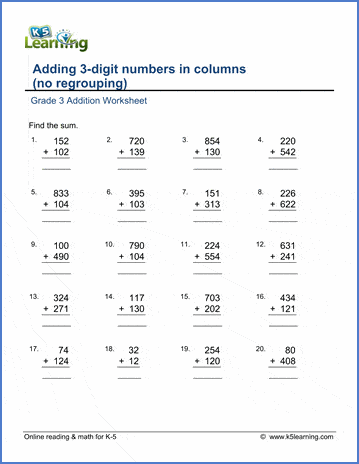## Free addition worksheets from K5 Learning

Our 3rd grade addition worksheets include both "mental addition problems" intended for students to solve in their heads and multi-digit column form addition questions giving practice in computational skills.

Example

Add 1-digit and 2-digit numbers   94 + 7 = ___
Add 1-digit and 2-digit numbers (missing addend)   94 + ___ = 103
Complete one hundred (missing addend)   ___ + 85 = 100

Adding a 2-digit and two 1-digit numbers   4 + 2 + 17 =
865 + 5 = ___

Whole tens
Add whole tens to 2-digit numbers   20 + 39 = ___
Add whole tens to 2-digit numbers (missing addend)   20 + ___ = 59

Adding two whole tens and two 1-digit numbers   70 + 10 + 8 + 6 =__
60 + 50 + 40 = ___
60+50+40+30= ___

Whole hundreds
300 + 200 = ___
Adding to complete whole thousands   7300 + ___ = 9000

Whole thousands:

Adding whole thousands and whole hundreds   7000 + 300 =
Adding whole thousands to another number   4,000 + 3,601 = ___

Adding two 2-digit numbers, no carrying

22

+ 23

38

+ 27

Adding two 3-digit numbers, no carrying

123

+ 234

216

+ 955

342

+ 123

+ 456

456

+ 123

+ 567

+ 890

5,432

+ 1,543

9,002

+ 1,004

+ 2,567

1,111

+ 2,222

+ 3,333

+ 4,444